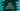# Numbers in Kotlin and different useful methods# Numbers in Kotlin and different useful methods:

Kotlin has 6 different built-in types that represents a number :

``````Double - 64 bit
Float - 32 bit
Long - 64 bit
Int  - 32 bit
Short - 16 bit
Byte - 8 bit``````

### How to declare a variable in Kotlin :

To declare a variable in Kotlin, we can either use var or val keyword. The only difference between var and val is that the variables declared using var are mutable , i.e. you can change their values but the variables declared using val are immutable, i.e. you can’t change the value. What is the output of the below example :

``````fun main(args: Array<String>) {
val number  = 12
print(number.javaClass.name)
}``````

It will print int. But what about :

``````fun main(args: Array<String>) {
val number: Byte = 12
print(number.javaClass.name)
}``````

it will print byte. So, we can also define the type of the variable.

### Inbuilt functions :

Following inbuilt functions are available in the Number class of kotlin :

### 1. toByte() :

toByte() converts the number to a Byte and returns the value.

### 2. toChar() :

toChar() converts the number to a Char, whose numeric value is equal to the number.

### 3. toDouble() :

toDouble() converts the number to a Double and returns it.

### 4. toFloat() :

It converts the number to Float value.

### 5. toInt() :

Converts the number to an Int and returns it.

### 6. toLong() :

Converts to a Long value and returns it.

### 7. toShort() :

Converts the number to a Short and returns it.

### 8. equals() :

It checks the equal between two numbers and returns a boolean value if both are equal or not.

### 9. hashCode() :

It returns the hash code of the number.

### 10. toString() :

Returns the string representation of the number object.

### Example :

``````fun main(args: Array<String>) {
val number1: Byte = -12
println("Type of number1 is "+ number1.javaClass.name)

val number2 = -12
println("Type of number2 is "+ number2.javaClass.name)

val number3:Short = -12
println("Type of number3 is "+ number3.javaClass.name)

val number4:Int = -12
println("Type of number4 is "+ number4.javaClass.name)

val number5:Long = 12
println("Type of number5 is "+ number5.javaClass.name)

val number6 = 12L
println("Type of number6 is "+ number6.javaClass.name)

val number7 = 12000000000
println("Type of number7 is "+ number7.javaClass.name)

val number8:Double = -12.13
println("Type of number8 is "+ number8.javaClass.name)

val number9 = 12.66
println("Type of number9 is "+ number9.javaClass.name)

val number10:Float = 11.66F
println("Type of number10 is "+ number10.javaClass.name)

val number11 = -12F
println("Type of number11 is "+ number11.javaClass.name)
}``````

### Output :

``````Type of number1 is byte
Type of number2 is int
Type of number3 is short
Type of number4 is int
Type of number5 is long
Type of number6 is long
Type of number7 is long
Type of number8 is double
Type of number9 is double
Type of number10 is float
Type of number11 is float``````